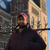# QlikView App Dev

Discussion Board for collaboration related to QlikView App Development.

Announcements
cancel
Showing results for
Did you mean:Creator II

Hello and happy New Year!
My current loading script uses some hard-coded date values to calculate some extra fields. My client added an extra table in his database to have the said date values be more dynamically modified, without having to modify the whole QlikView loading script. Now my issue is, how can I extract the values from the said table and use them in the loading script?
For the record the hard-coded date values look kind of like this:

LET Cycle1_start = MakeDate(2017,01,10);

Cycle1_stop = MakeDate(2017,04,28);

Cycle2_start = MakeDate(2017,05,02);

Cycle2_stop=MakeDate(2017,09,11);

Cycle3_start=MakeDate(2017,09,12);

Cycle3_stop=MakeDate(2018,01,08);

The "Cycle" table has the following structure:

`id` bigint(20) NOT NULL AUTO_INCREMENT,

`version` bigint(20) NOT NULL,

`date_end` datetime NOT NULL,

`date_start` datetime NOT NULL,

`deleted` bit(1) NOT NULL,

`name` varchar(255) NOT NULL,

PRIMARY KEY (`id`)

ENGINE=InnoDB AUTO_INCREMENT=8 DEFAULT CHARSET=latin1;

1 Solution

Accepted SolutionsSpecialist

Hi,

You could use the peek function to find the records and fields you need. The below is just an example that assumes the first 3 records have the corresponding cycles...

Cycle:

from cycle

where etc...

order by ...;

Let Cycle1_start = Date(Peek('date_start', 0, 'Cycle'));

Let Cycle1_stop = Date(Peek('date_stop', 0, 'Cycle'));

Let Cycle2_start = Date(Peek('date_start', 1, 'Cycle'));

Let Cycle2_stop = Date(Peek('date_stop', 1, 'Cycle'));

Let Cycle3_start = Date(Peek('date_start', 2, 'Cycle'));

Let Cycle3_stop = Date(Peek('date_stop', 2, 'Cycle'));

Drop Table Cycle;

I hope this helps,

Cheers,

Luis

2 RepliesChampion

Hi,

let vDate_end = peek ( 'date_end', 0 , ' the_name_of_your_loaded_table ');

you will have your Date end value stored into the variable and you can use it wherever you want on the scriptSpecialist

Hi,

You could use the peek function to find the records and fields you need. The below is just an example that assumes the first 3 records have the corresponding cycles...

Cycle:

from cycle

where etc...

order by ...;

Let Cycle1_start = Date(Peek('date_start', 0, 'Cycle'));

Let Cycle1_stop = Date(Peek('date_stop', 0, 'Cycle'));

Let Cycle2_start = Date(Peek('date_start', 1, 'Cycle'));

Let Cycle2_stop = Date(Peek('date_stop', 1, 'Cycle'));

Let Cycle3_start = Date(Peek('date_start', 2, 'Cycle'));

Let Cycle3_stop = Date(Peek('date_stop', 2, 'Cycle'));

Drop Table Cycle;

I hope this helps,

Cheers,

Luis